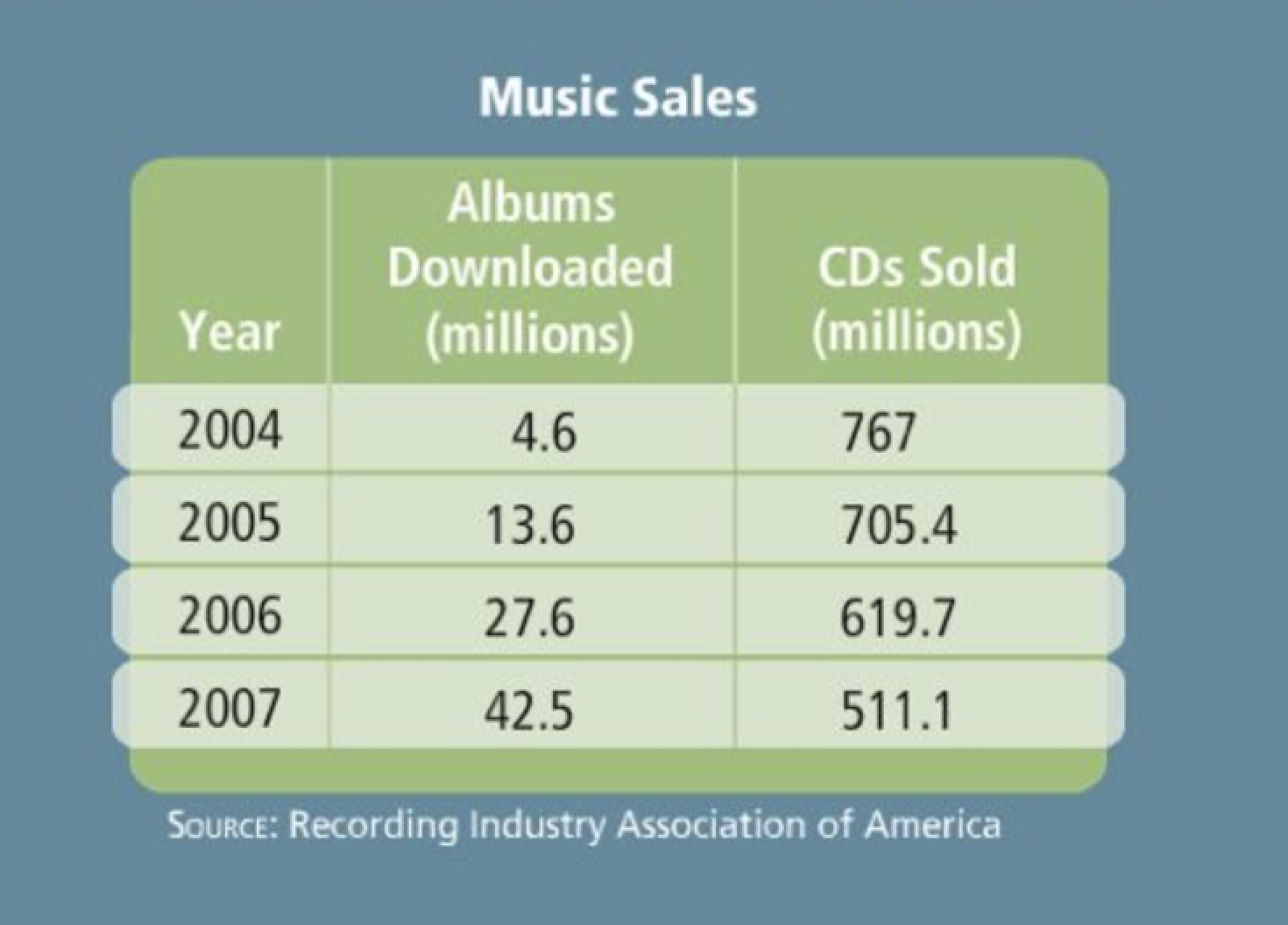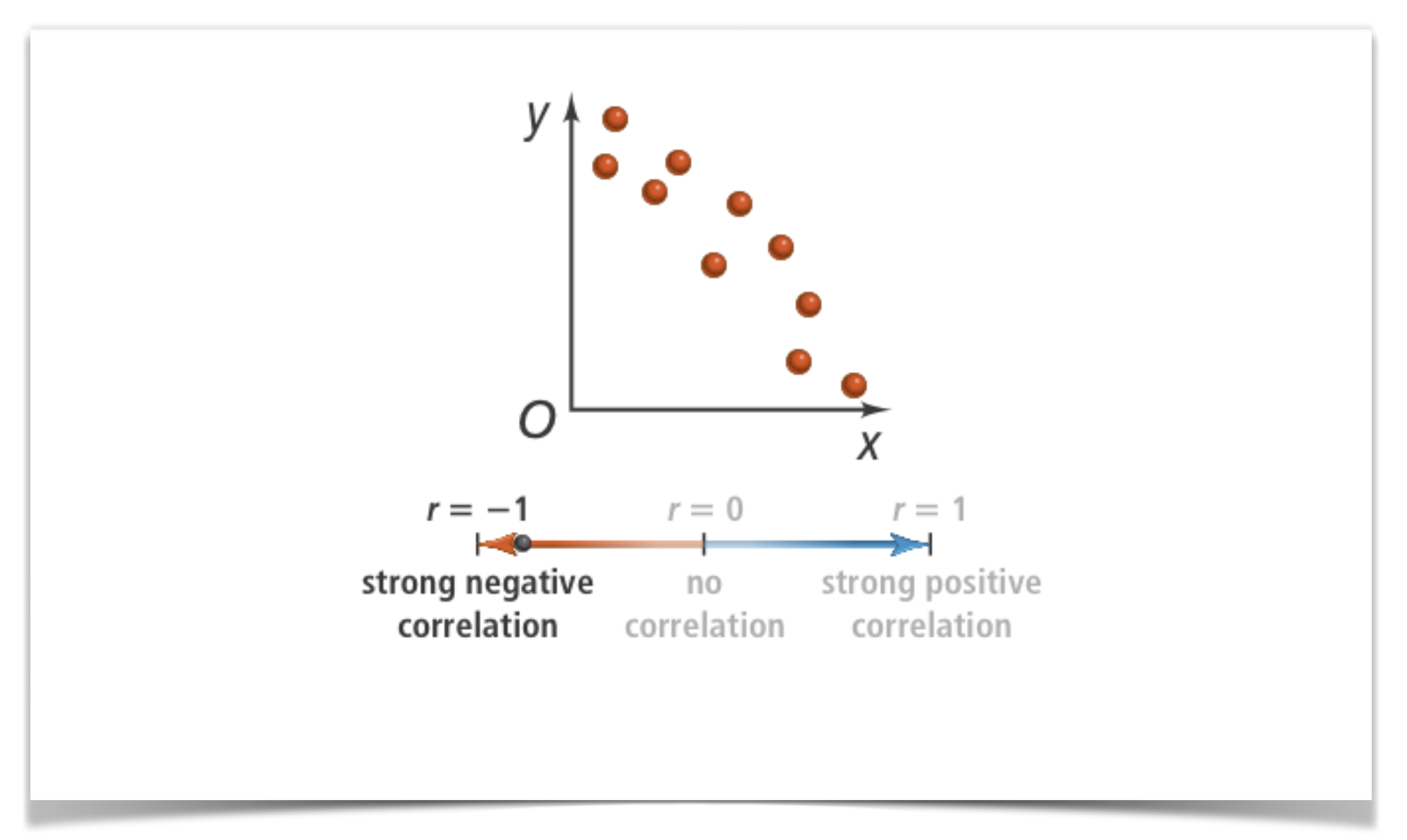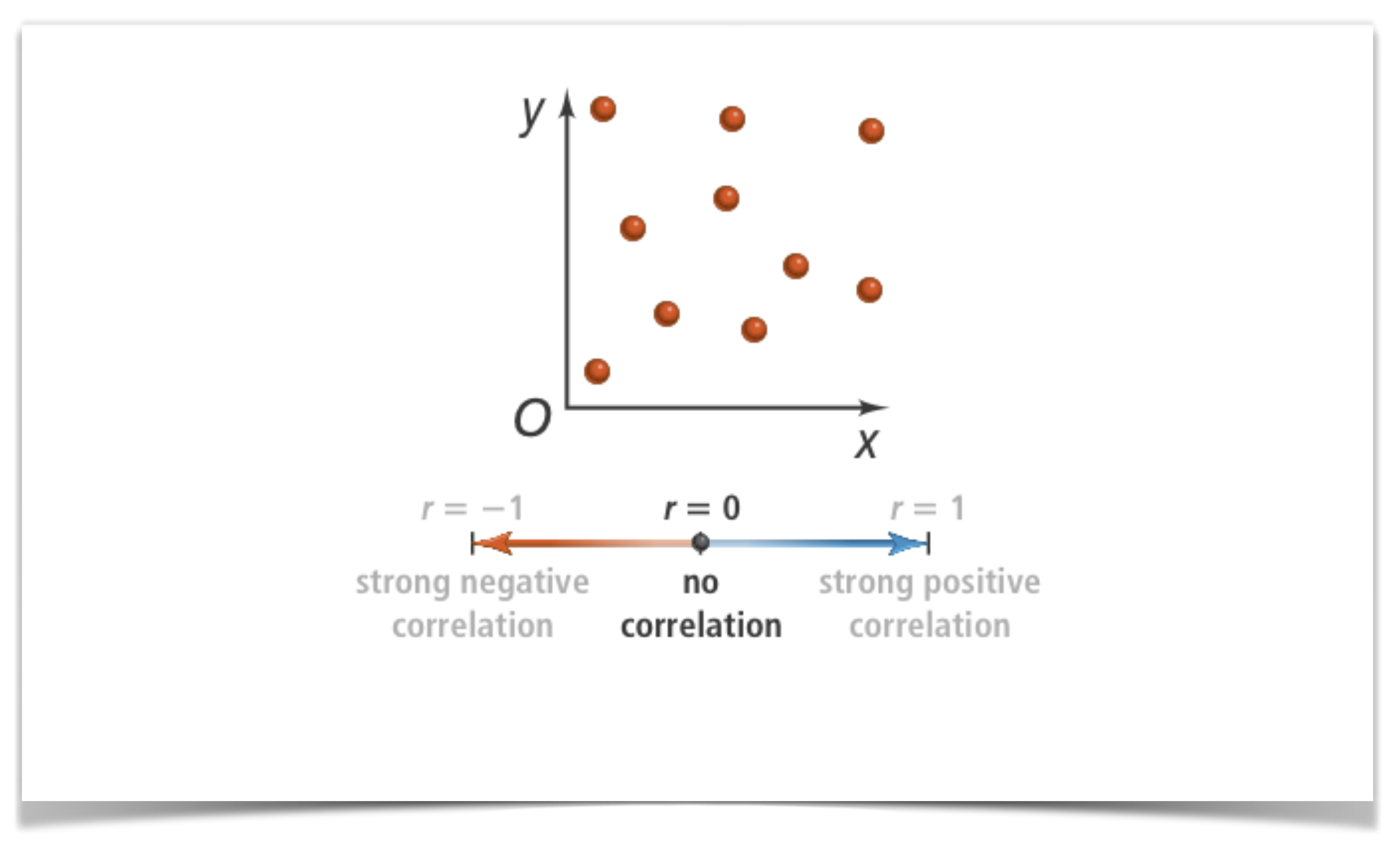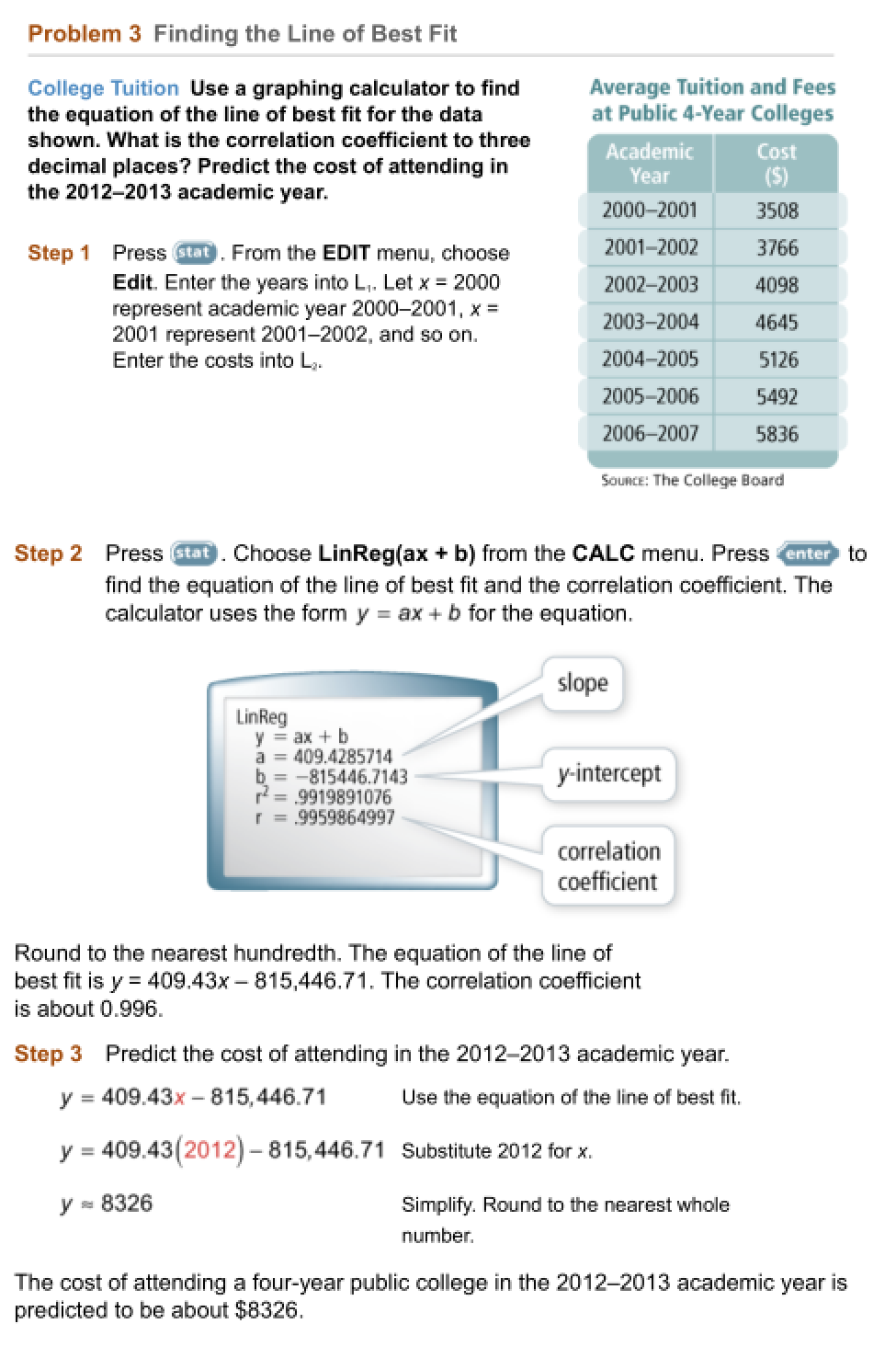Algebra 1 5-7 Complete Lesson: Scatter Plots and Trend Lines
starstarstarstarstarstarstarstarstarstar
4.5 (1 rating)
by Matthew Richardson
| 27 Questions
Note from the author:
A complete formative lesson with embedded slideshow, mini lecture screencasts, checks for understanding, practice items, mixed review, and reflection. I create these assignments to supplement each lesson of Pearson's Common Core Edition Algebra 1, Algebra 2, and Geometry courses. See also mathquest.net and twitter.com/mathquestEDU.The outlined content above was added from outside of Formative.1
2
1
10 pts
Solve It! The table shows the number of digital albums downloaded per year and the number of CDs sold by manufacturers per year. What relationship exists between the two sets of data?
2
10 pts
Solve It! Predict the number of CDs sold and the number of albums downloaded in 2010. Explain your reasoning.3
10 pts
Problem 1 Got It? Make a scatter plot of the data in the table.
Be sure to include relevant graph detail: label axes, indicate units and scale on both axes, and use arrows to represent end behavior, as appropriate.
4
10 pts
Problem 1 Got It? What type of relationship does the scatter plot you created in the previous item show?
positive correlation
negative correlation
no correlation6
10 pts
Problem 2 Got It? Make a scatter plot of the data in the table. Draw a trend line and write its equation.

Be sure to include relevant graph detail: label axes, indicate units and scale on both axes, and use arrows to represent end behavior, as appropriate.
7
10 pts
Problem 2 Got It? Use the trend line you drew in the previous item to predict the approximate body length of a 7-month-old panda.The outlined content above was added from outside of Formative.8
9
8
10 pts
Problem 3 Got It? Use the equation of the line of best fit from Problem 3 to predict the cost of attending in the 2016-2017 academic year. You may use the embedded Desmos 4-function calculator above.

Include a dollar sign and round your answer to the nearest whole dollar.
9
10 pts
Problem 3 Got It? Reasoning: What does the slope of the line of best fit in Problem 3 tell you about the rate of change in the cost?10
10 pts
Problem 4 Got It? In the following situation, is there likely to be a correlation? If so, does the correlation reflect a causal relationship? Explain.

the cost of a family's vacation and the size of their house
11
10 pts
Problem 4 Got It? In the following situation, is there likely to be a correlation? If so, does the correlation reflect a causal relationship? Explain.

the time spent exercising and the number of Calories burned12
20 pts
Make a scatter plot of the data, draw an appropriate trend line, and write the equation of the trend line.
Be sure to include relevant graph detail: label axes, indicate units and scale on both axes, and use arrows to represent end behavior, as appropriate.
13
10 pts
Consider your scatter plot and the data in the previous item. What type of relationship is shown?
Strong positive correlation
Positive correlation
Little to no correlation
Negative correlation
Strong negative correlation
14
10 pts
Consider your scatter plot and the data once more. Predict the average maximum daily temperature in January at a latitude of 50° N.
Choose the most reasonable prediction.15
10 pts
Vocabulary: Given a set of data pairs, how would you decide whether to use interpolation or extrapolation to find a certain value?
16
10 pts
Compare and Contrast How are a trend line and the line of best fit for a set of data pairs similar? How are they different?1718
10 pts
Review Lesson 5-6: Write an equation of the line in slope-intercept form that passes through the given point and is parallel to the graph of the given equation.

y + 3 = 5(x - 2)
y = 5x - 13
y = -2x + 3
19
10 pts
Review Lesson 5-6: Write an equation of the line in slope-intercept form that passes through the given point and is parallel to the graph of the given equation.

y = -x + 5
y = x + 9
y = 5x
20
5 pts
Review Lesson 1-5: Find the absolute value.
Enter only a number.
21
5 pts
Review Lesson 1-5: Find the absolute value.
Enter only a number.
22
5 pts
Review Lesson 1-5: Find the absolute value.
Enter only a number.
23
5 pts
Review Lesson 1-5: Find the absolute value.
Enter only a number.24
10 pts
Vocabulary Review: A scatter plot is a graph that relates two sets of data. Plot each ordered pair on the graph on the canvas to make a scatter plot.25
10 pts
Use Your Vocabulary: Identify the correlation shown in each scatter plot above.
• positive correlation
• negative correlation
• no correlation
• Scatter Plot A
• Scatter Plot B
• Scatter Plot C
26
100 pts
Notes: Take a clear picture or screenshot of your Cornell notes for this lesson. Upload it to the canvas. Zoom and pan as needed.

For a refresher on the Cornell note-taking system, click here.
27
10 pts
Reflection: Math Success
Add to my formatives list

Formative uses cookies to allow us to better understand how the site is used. By continuing to use this site, you consent to the Terms of Service and Privacy Policy.